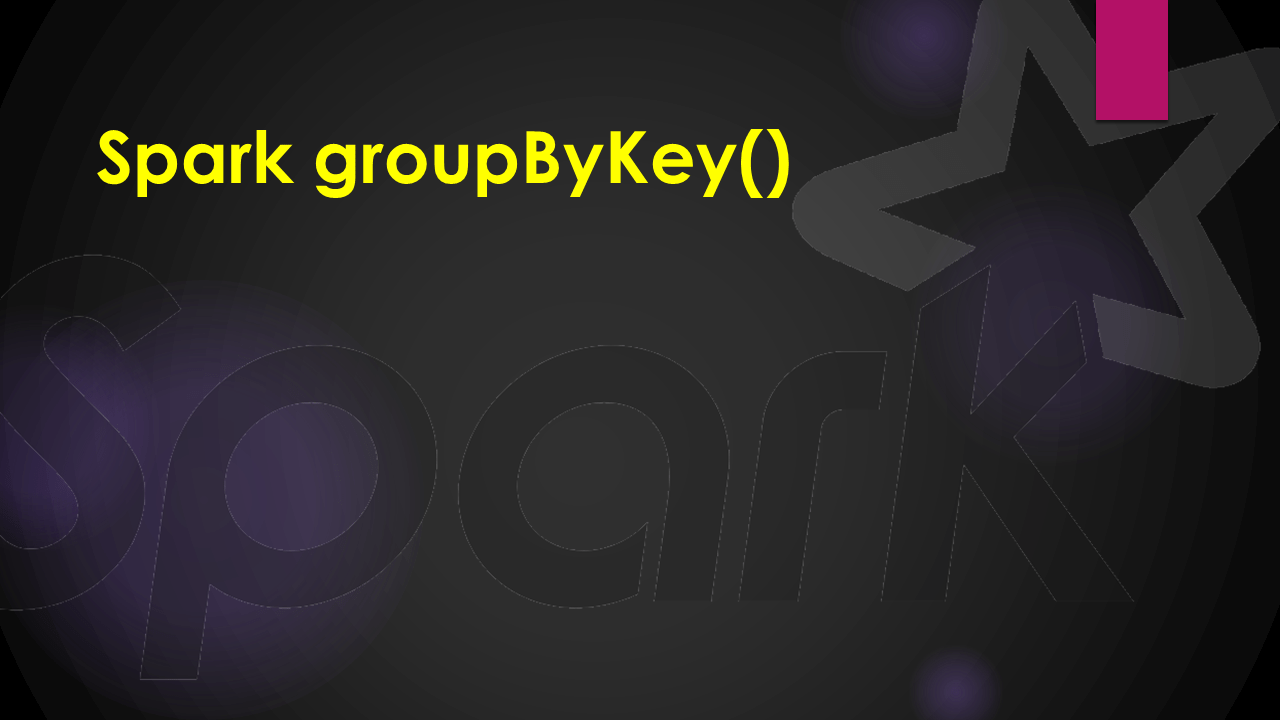# Spark groupByKey()

The Spark or PySpark `groupByKey()` is the most frequently used wide transformation operation that involves shuffling of data across the executors when data is not partitioned on the Key. It takes key-value pairs (K, V) as an input, groups the values based on the key(K), and generates a dataset of `KeyValueGroupedDataset`(K, Iterable) pairs as an output.

It’s a very expensive operation and consumes a lot of memory if the dataset is huge.

Now let us see how we can implement the Spark groupByKey() function on an RDD with an example

## 1. Spark groupByKey()

``````
// Create an RDD
val rdd = spark.sparkContext.parallelize(Seq(("A",1),("A",3),("B",4),("B",2),("C",5)))
// Get the data in RDD
val rdd_collect = rdd.collect()

// Output:
rdd_collect: Array[(String, Int)] = Array((A,1), (A,3), (B,4), (B,2), (C,5))
``````

Above we have created an RDD which represents an Array of (name: String, count: Int) and now we want to group those names using Spark groupByKey() function to generate a dataset of Arrays for which each item represents the distribution of the count of each name like this (name, (id1, id2) is unique).

``````
// Group rdd based on key
rdd.groupByKey.collect()

// Output:
res15: Array[(String, Iterable[Int])] = Array((A,CompactBuffer(1, 3)), (B,CompactBuffer(4, 2)), (C,CompactBuffer(5)))

``````

Note: Spark groupByKey() method is recommended when there are no required aggregation over each key.

## 2. Compare groupByKey vs reduceByKey

When we work on large datasets, reduceByKey() function is more preffered when compared with Spark groupByKey()` `function. Let us check it out with an example.

``````
// Using ReduceByKey() function
val nameCountsWithReduce = rdd
.reduceByKey(_ + _)
.collect()

// Using GroupByKey() function
val nameCountsWithGroup = rdd
.groupByKey()
.map(x => (x._1, x._2.sum))
.collect()

// Output:
nameCountsWithReduce: Array[(String, Int)] = Array((A,4), (B,6), (C,5))
nameCountsWithGroup: Array[(String, Int)] = Array((A,4), (B,6), (C,5))
``````

From the above example, it is clear that the output of both functions results in the same whereas reduceByKey() works much better on a large dataset when compared to the Spark groupByKey() function. The main reason for the performance difference is that reduceByKey() results in less shuffling of data as Spark knows it can combine output with a common key on each partition before shuffling the data.

Look at the below diagram to understand what happens when we use reduceByKey() vs Spark groupByKey() on our example dataset.

From the above diagram, when calling Spark `groupByKey` on the dataset,

• All the key-value pairs are shuffled across the executors.
• This is a lot of unnecessary data to be transferred over the network.
• Spark uses the partition function on the dataset to determine which partition to be shuffled across the executors.
• Spark `groupByKey` spills data to disk when there is more data shuffled onto a single executor machine than can fit in memory.
• If the size of the data is more than the memory of the executor Spark spills out the data to disk one key at a time – so if a single key has more key-value pairs than can fit in executor memory, an out-of-memory exception occurs.
• This was handled in a later release of Spark so the job can still proceed, but should still be avoided – when Spark needs to spill to disk, performance is severely impacted.

Whereas when we use `reduceByKey` on the same datasets,

• key-value pairs on the same machine with the same key are combined (by using the lambda function passed into `reduceByKey` ) before the data is shuffled.
• The lambda function is called again to reduce all the values from each partition to produce one final result.
• In this way, shuffling is minimal in the case of ReduceByKey and improvises performance a lot faster.

## 3. Conclusion

Both Spark groupByKey() and reduceByKey() are part of the wide transformation that performs shuffling at some point each. The main difference is when we are working on larger datasets reduceByKey is faster as the rate of shuffling is less than compared with Spark groupByKey(). We can also use combineByKey() and foldByKey() as a replacement to groupByKey()

### rimmalapudi

Data Engineer. I write about BigData Architecture, tools and techniques that are used to build Bigdata pipelines and other generic blogs.